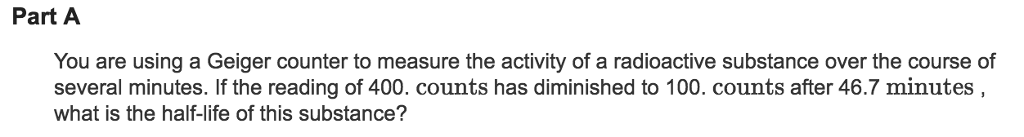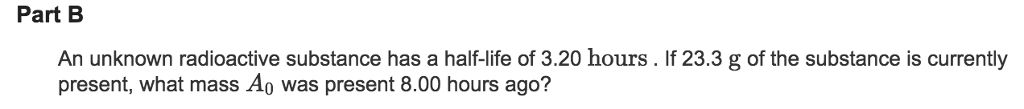# Question & Answer: Part A You are using a Geiger counter to measure the activity of a radioactive substance over…..Both parts please!! How to approach!!

Part A You are using a Geiger counter to measure the activity of a radioactive substance over the course of several minutes. If the reading of 400. counts has diminished to 100. counts after 46.7 minutes what is the half-life of this substance?

Part A

Don't use plagiarized sources. Get Your Custom Essay on
Question & Answer: Part A You are using a Geiger counter to measure the activity of a radioactive substance over…..
GET AN ESSAY WRITTEN FOR YOU FROM AS LOW AS \$13/PAGE

400 counts dropping to 100 counts means two half lives have passed

400 –> 200 one half life
200 –> 100 one half life

so the half life is 46.7/2 = 23.35 mins

Part B

Use this equation below to find out how much of a radioactive material is left after a given period of time. You can use the equation in reverse to find out the initial
mass of the radioactive material if you know its current mass.

A = current mass of radioactive material = 23.3 g
A0 = initial mass of radioactive material = to be determined
T½ = half-life of the radioactive material = 3.20 h
t = elapsed time since the original mass was present = 8.00 h

A = A0e^(-0.693t/T½)
A/A0 = e^(-0.693t/T½)
ln (A/A0) = ln [e^(-0.693t/T½)]
ln A – ln A0 = (-0.693t/T½)
ln A = -0.693t/T½ + ln A0
ln 23.3 = -0.693(8.00 h)/3.20 h + ln A0
3.1485 = -1.7325 + ln A0
3.1485 + 1.7325 = ln A0
ln A0 = 3.1485 + 1.7325
ln A0 = 4.881
A0 = e^4.881
A0 = 131.76 g Initial Mass of Radioactive Material